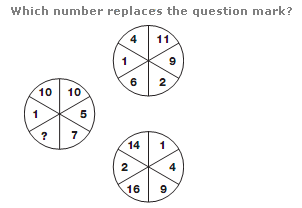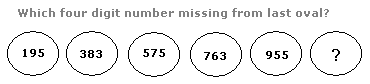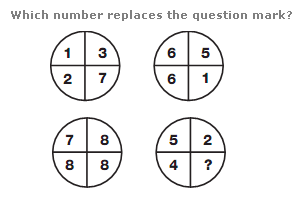# Puzzles - Number puzzles

### Exercise :: Number puzzlesAnswer : 10 Explanation : Numbers in the segments of the left hand circle equal the difference between numbers in corresponding segments of the two right hand circles.Answer : 1,143 Explanation : Starting with the top 3 digit number, the first digit increases by 2 as you descend, from 1 to 11. The middle digit decreases by 1 each time, and the right hand digit alternates between 5 and 3.Answer : 6 Explanation : Numbers in the lower left circle equal the sum of the numbers in corresponding segments of the top two circles, and numbers in the lower right circle equal the difference of the numbers in corresponding segments of the top two circles.